Question

Coordinate plane with quadrilaterals EFGH and E prime F prime G prime H prime at E 0 comma 1, F 1 comma 1, G 2 comma 0, H 0 comma 0, E prime negative 1 comma 2, F prime 1 comma 2, G prime 3 comma 0, and H prime negative 1 comma 0. F and H are connected by a segment, and F prime and H prime are also connected by a segment. Quadrilateral EFGH was dilated by a scale factor of 2 from the center (1, 0) to create E’F’G’H’. Which characteristic of dilations compares segment F’H’ to segment FH

1.RobertKer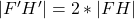Step-by-step explanation:

Given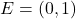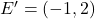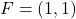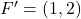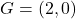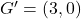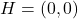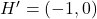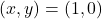— center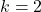— scale factor

See comment for proper format of question

Required

Compare FH to F’H’

From the question, we understand that the scale of dilation from EFGH to E’F’G’H is 2;

Irrespective of the center of dilation, the distance between corresponding segment will maintain the scale of dilation.

i.e.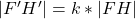To prove this;

Calculate distance of segments FH and F’H’ using: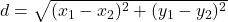Given that:We have: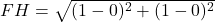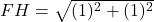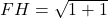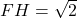Similarly;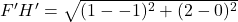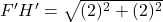Distribute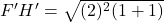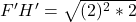Split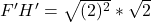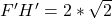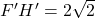Recall that:So, we have: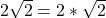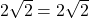— true

Hence, the dilation relationship between FH and F’H’ is::